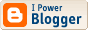Fixing Figure References

My client assured me that figure references in a particular kind of feature box used in the chapters of the book I'm in the process of producing would always be in the form "Fig3-1" where "3" is the chapter number and "1" is the figure number. The figures themselves are in files named Fig3-1.psd or .tif or .pdf or even .eps.

So, I wrote a script to autmatically import the figures based on what I found in the relevant paragraphs. But then chapters started arriving with the references in this form:

Fig3-1: Description of figure

or:

<Fig3-1: Description of figure>

or:

<Figure 3-1: Description of figure>

So, rather than just take the contents of the paragraph and use that to build the file name, I had to filter the contents to get the name of file. Here's the function I'm currently using that deals with the above cases:
function stripName(theName) {
theName = theName.split("Figure ").join("Fig");
var theStart = theName.indexOf("Fig");
var theEnd = theName.indexOf(":");
if ((theStart == 0) && (theEnd == -1)) return theName;
return theName.slice(theStart,theEnd)
}
Even as I prepared this note, it occurs to me that this function doesn't deal with the format:

<Fig3-1>

You can bet your life I'll be getting some of those soon enough. I guess the easy way to deal with that is to add a test to see if theEnd contains -1, and if so, do a search for ">". This should do it:
function stripName(theName) {
theName = theName.split("Figure ").join("Fig");
var theStart = theName.indexOf("Fig");
var theEnd = theName.indexOf(":");
if(theEnd == -1) { theEnd = theName.indexOf(">") }
if ((theStart == 0) && (theEnd == -1)) return theName;
return theName.slice(theStart,theEnd)
}
So, if the colon is absent, we check for a closing angle bracket, and if that's there use it for the end of the slice we take out of the text provided in the call. Of course, if the client manages to forget the colon but still includes the description, I'm going to end up with the wrong name, but that's not a disaster. The way I have the main routine structured, if it can't find the file, it puts the name of the (presumed) missing file into the frame instead of the image, so that missing files are not only easy to see on the page, I also know which one is missing.